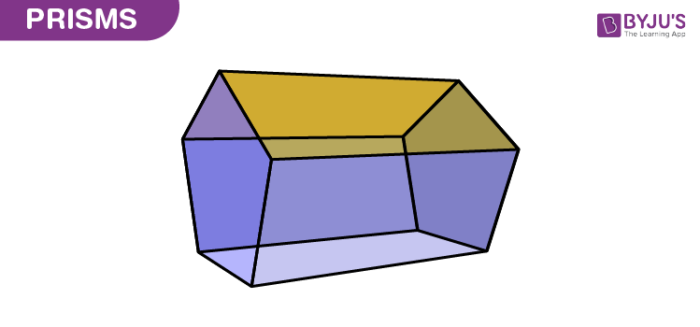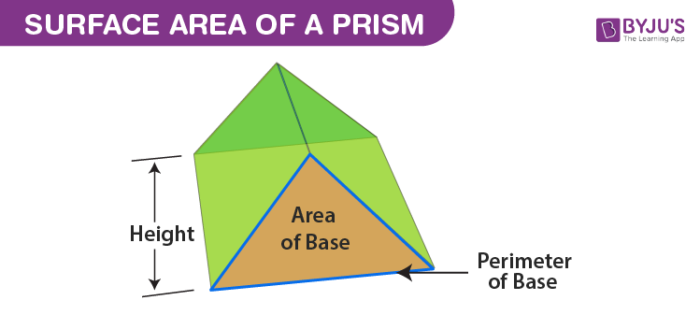# Prism

Prism is a three-dimensional solid object in which the two ends are identical. It is the combination of the flat faces, identical bases and equal cross-sections. The faces of the prism are parallelograms or rectangles without the bases. And the bases of the prism could be triangle, square, rectangle or any n-sided polygon. For example, a pentagonal prism has two pentagonal bases and 5 rectangular faces.

## Prism Shape

A prism has a solid shape consisting of two identical ends (such as triangle, square, rectangle, etc.), flat faces or surfaces and uniform cross-section across its length. The cross-section looks like a triangle hence called triangular prism. The shape of the prism does not have any curve. Therefore, a prism can have square, rectangular, pentagonal and other polygon shapes but not the circular shape.Also, check:

## Cross Section of Prism

The cross-section is the shape obtained by the intersection of an object by a plane along its axis. It is also said as cutting a three-dimensional object with a plane to obtain another shape.

If a prism is intersected by a plane, parallel to the base, then the shape of cross-section will be same as the base. For instance, a square pyramid is cut by a plane, parallel to the base, then the shape of cross-section of pyramid will also be a square.

## Types of Prism

Depending upon the cross-sections, the prisms are named. It is of two types, namely;

• Regular Prism
• Irregular Prism

### Regular Prism

If the bases of the prism are in the shape of a regular polygon, it is called regular prism.

### Irregular Prism

If the bases are in the shape of an irregular polygon, then the prism is called an irregular prism.

### Prism Based on Shape of Bases

Based on the shape of the bases, it is further categorised into different types, namely;

• Triangular prism (has triangular bases)
• Square prism (has square bases)
• Rectangular prism (has rectangular bases)
• Pentagonal prism (has pentagonal bases)
• Hexagonal prism (has hexagonal bases)

## Right Prism And Oblique Prism

Apart from regular and irregular, the prism can be classified into two more types;

• Right Prism
• Oblique Prism

The difference between both the prism for triangular bases are;

 Right Prism Oblique Prism If the faces and the joining edges are perpendicular to the base faces, then it is known as right prism If the faces and the joining edges are not perpendicular to the base faces, then it is known as oblique prism In a right prism, the side faces are rectangles In an oblique prism, the side faces are parallelograms Surface area = [Base length x height] + 2[prism length x side length]+[prism length x base length] Surface area = [Base length x height] + 2[prism length x side length]+[prism length x base length] Volume = ½ [base length x height x prism length] Volume = ½ [base length x height x prism length]

## Formulas (Surface Area & Volume)

The formulas are defined for the surface area and volume of the prism. As the prism is a three-dimensional shape, so it has both the properties, i.e., surface area and volume.

### Surface Area of a Prism

The surface area of the prism is the total area covered by the faces of the prism.For any kind of prism, the surface area can be found using the formula;

 Surface Area of a Prism = 2(Base Area)+ (Base perimeter × height)

### Volume of a Prism

The volume of the prism is defined as the product of the base area and the prism height.

Therefore,

 Volume of Prism = Base Area × Height

For example, if you want to find the volume of a square prism, you must know the area of a square, then its volume can be calculated as follows:

The volume of a square Prism = Area of square×height

V = s2 × h cubic units

Where “s” is the side of a square.

## Solved Problems

Example 1: Find the volume of a triangular prism whose area is 60 cm2 and height is 7 cm.

Solution: Given,

Base area = 60 cm2

Height = 7 cm

We know that,

The volume of a prism = (Base area × Height) cubic units

Therefore, V = 60 ×7 = 420

Hence, the volume of a triangular prism = 420 cm3.

Example 2: Find the height of the square prism whose volume is 360 cm3 and the base area is 60 cm2.

Solution: Given,

The volume of a square prism = 360 cm3

Base Area = 60 cm2

Therefore, the height of the square prism is calculated as follows:

The volume of square prism = Base area × height

360 = 60 × prism height

Therefore, the height, h = 360/60

Prism Height, h = 6 cm.

For more information on other maths-related articles, stay tuned with BYJU’S – The Learning App and also watch interactive videos to learn with ease.

## Frequently Asked Questions – FAQs

### What is Prism?

A prism is a three dimensional solid, that has two identical bases, rectangular or parallelogram-shaped faces and same cross-section.

### What are the examples of Prism?

Based on the shapes of bases of a Prism, we have triangular prism, square prism, rectangular prism, pentagonal prism, hexagonal prism.

### What is the difference between prism and pyramid?

Both prism and pyramid are three dimensional solids that have flat-faces and base. But a Prism has two identical bases whereas a pyramid has only one base.

### What is the type of Prism?

The prism is majorly divided into two categories: Regular and irregular.
A regular prism has regular polygons as their bases, i.e. the triangular prism will have equilateral triangle bases, a square prism will have square bases.
An irregular prism will have irregular polygons as their bases.

### What is the cross section of prism?

The cross section of prism is the shape obtained when the prism is intersected by a plane. If the prism is cut by a plane horizontally, parallel to the base, then the shape of cross section will be equal to the shape of base.

#### 1 Comment

1. Juliet Ojoma Ogah

Wow this is a great website my daughter is learning so much about it. Like:Cylinder Properties
Some of the important properties of the cylinder are as follows:

The bases of the cylinder are always congruent and parallel to each other.
If the axis of the cylinder is a right angle to the base and the bases are exactly over each other, then it is called as “Right Cylinder”.
If one of the bases of the cylinder is displayed sideways, and the axis does not produce the right angle to the bases, then it is called “Oblique Cylinder”.
If the bases are circular, then it is called a right circular cylinder.
The best alternative to the circular base of a cylinder is an ellipse. If the base of the cylinder is elliptical in shape, then it is called an “Elliptical Cylinder”.
If the locus of a line moving parallel and fixed distance from the axis, a circular cylinder is produced.
A cylinder is similar to the prism since it has the same cross-section everywhere.